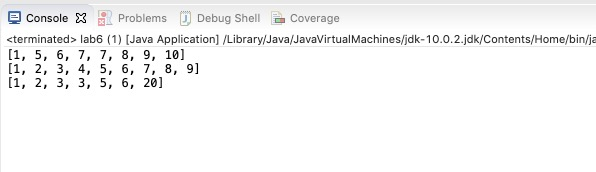# Lab 5

Today in lab we're going to fix the quicksort algorithum I created! I created this very buggy version of quicksort and it's you and your teams job to fix it! We'll start class by going over the debugger quickly and you should be able to use that to fix the code I gave you!

• ``````package labs;
public class lab6 {
public static void main(String args[])
{
int arr[] = {10, 7, 8, 9, 1, 5,6,7};
int arr2[] = {9, 8, 7, 6, 5, 4, 3, 2, 1};
int arr3[] = {1, 3, 5, 3, 2, 6, 20};
quicksort(arr,0,arr.length-1);
quicksort(arr2,0,arr2.length-1);
quicksort(arr3,0,arr3.length-1);
System.out.println(Arrays.toString(arr));
System.out.println(Arrays.toString(arr2));
System.out.println(Arrays.toString(arr3));

}
}``````

Here is the code to get started:

• ``````private static int partition(int[] items,int low, int high)
{

int i=0;
int j=0;
int k=high+1;
int pivot=0;
while(j < k)
{
if (items[j] < pivot)
{
swap(j,i,items);
j++;
i++;
}
else if(items[j]==pivot)
{
j++;
}
else
{
swap(j,k-1,items);
k--;
}
}
return i;
}

private static void swap(int i,int j,int[] items)
{
int temp=items[i];
items[i]=items[j];
items[j]=temp;
}

public static void quicksort(int[] arr)
{
quicksort(arr,0,arr.length-1);
}
private static void quicksort(int[] arr, int low, int high)
{
if (low < high)
{
/* pi is partitioning index, arr[pi] is
now at right place */
int pi = partition(arr,low,high);

// Recursively sort elements before
// partition and after partition
quicksort(arr, low, pi-1);
quicksort(arr, pi+1, high);
}
}  ``````

NOTE: I am asking you to create an object oriented approach to this problem. You will have to think about that as you formulate your solution.

Here are some resources to get you started:

You should get this as an output: